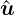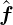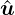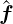### 2.2 Methods overview

Here a review of some terminology in order to situate the spectral/hp element method within the field of the finite element methods.

#### 2.2.1 The finite element method (FEM)

Nowadays, the finite element method is one of the most popular numerical methods in the field of both solid and fluid mechanics. It is a discretisation technique used to solve (a set of) partial differential equations in its equivalent variational form. The classical approach of the finite element method is to partition the computational domain into a mesh of many small subdomains and to approximate the unknown solution by piecewise linear interpolation functions, each with local support. The FEM has been widely discussed in literature and for a complete review of the method, the reader is also directed to the seminal work of Zienkiewicz and Taylor .

#### 2.2.2 High-order finite element methods

While in the classical finite element method the solution is expanded in a series of linear basis functions, high-order FEMs employ higher-order polynomials to approximate the solution. For the high-order FEM, the solution is locally expanded into a set of P + 1 linearly independent polynomials which span the polynomial space of order P. Confusion may arise about the use of the term order. While the order, or degree, of the expansion basis corresponds to the maximal polynomial degree of the basis functions, the order of the method essentially refers to the accuracy of the approximation. More specifically, it depends on the convergence rate of the approximation with respect to mesh-refinement. It has been shown by Babuska and Suri , that for a sufficiently smooth exact solution u ∈ Hk(Ω), the error of the FEM approximation uδ can be bounded by:

 ||u - uδ|| E ≤ ChP ||u|| k.

This implies that when decreasing the mesh-size h, the error of the approximation algebraically scales with the Pth power of h. This can be formulated as:

 ||u - uδ|| E = O(hP ).

If this holds, one generally classifies the method as a Pth-order FEM. However, for non-smooth problems, i.e. k < P + 1, the order of the approximation will in general be lower than P, the order of the expansion.

##### 2.2.2.1 h-version FEM

A finite element method is said to be of h-type when the degree P of the piecewise polynomial basis functions is fixed and when any change of discretisation to enhance accuracy is done by means of a mesh refinement, that is, a reduction in h. Dependent on the problem, local refinement rather than global refinement may be desired. The h-version of the classical FEM employing linear basis functions can be classified as a first-order method when resolving smooth solutions.

##### 2.2.2.2 p-version FEM

In contrast with the h-version FEM, finite element methods are said to be of p-type when the partitioning of domain is kept fixed and any change of discretisation is introduced through a modification in polynomial degree P. Again here, the polynomial degree may vary per element, particularly when the complexity of the problem requires local enrichment. However, sometimes the term p-type FEM is merely used to indicated that a polynomial degree of P > 1 is used.

##### 2.2.2.3 hp-version FEM

In the hp-version of the FEM, both the ideas of mesh refinement and degree enhancement are combined.

##### 2.2.2.4 The spectral method

As opposed to the finite element methods which builds a solution from a sequence of local elemental approximations, spectral methods approximate the solution by a truncated series of global basis functions. Modern spectral methods, first presented by Gottlieb and Orzag , involve the expansion of the solution into high-order orthogonal expansion, typically by employing Fourier, Chebyshev or Legendre series.

##### 2.2.2.5 The spectral element method

Patera  combined the high accuracy of the spectral methods with the geometric flexibility of the finite element method to form the spectral element method. The multi-elemental nature makes the spectral element method conceptually similar to the above mentioned high-order finite element. However, historically the term spectral element method has been used to refer to the high-order finite element method using a specific nodal expansion basis. The class of nodal higher-order finite elements which have become known as spectral elements, use the Lagrange polynomials through the zeros of the Gauss-Lobatto(-Legendre) polynomials.

##### 2.2.2.6 The spectral/hp element method

The spectral/hp element method, as its name suggests, incorporates both the multi-domain spectral methods as well as the more general high-order finite element methods. One can say that it encompasses all methods mentioned above. However, note that the term spectral/hp element method is mainly used in the field of fluid dynamics, while the terminology p and hp-FEM originates from the area of structural mechanics.

#### 2.2.3 The Galerkin formulation

Finite element methods typically use the Galerkin formulation to derive the weak form of the partial differential equation to be solved. We will primarily adopt the classical Galerkin formulation in combination with globally C0 continuous spectral/hp element discretisations.

To describe the Galerkin method, consider a steady linear differential equation in a domain Ω denoted by

 L(u) = f,

subject to appropriate boundary conditions. In the Galerkin method, the weak form of this equation can be derived by pre-multiplying this equation with a test function v and integrating the result over the entire domain Ω to arrive at: Find u ∈ U such that

 ∫ ΩvL(u)dx = ∫ Ωvfdx, ∀v ∈V,

where U and V respectively are a suitably chosen trial and test space (in the traditional Galerkin method, one typically takes U = V). In case the inner product of v and L(u) can be rewritten into a bi-linear form a(v,u), this problem is often formulated more concisely as: Find u ∈U such that

 a(v,u) = (v,f), ∀v ∈V,

where (v,f) denotes the inner product of v and f. The next step in the classical Galerkin finite element method is the discretisation: rather than looking for the solution u in the infinite dimensional function space U, one is going to look for an approximate solution uδ in the reduced finite dimensional function space Uδ ⊂ U. Therefore we represent the approximate solution as a linear combination of basis functions Φn that span the space Uδ, i.e.

 uδ = ∑ n∈NΦnûn.

Adopting a similar discretisation for the test functions v, the discrete problem to be solved is given as: Find ûn (n ∈N) such that

 ∑ n∈Na(Φm,Φn)ûn = (Φm,f), ∀m ∈N.

It is customary to describe this set of equations in matrix form as

 A=,

whereis the vector of coefficients ûn, A is the system matrix with elements

 A[m][n] = a(Φm,Φn) = ∫ ΩΦmL(Φn)dx,

and the vectoris given by[m] = (Φm,f) = ∫ ΩΦmfdx.